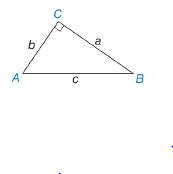Chapter 3.2, Problem 25E### Elementary Geometry for College St...

6th Edition
Daniel C. Alexander + 1 other
ISBN: 9781285195698

#### Solutions

Chapter
Section### Elementary Geometry for College St...

6th Edition
Daniel C. Alexander + 1 other
ISBN: 9781285195698
Textbook Problem
1 views

# In Exercise 21 to 26, Δ A B C is a right triangle. Use the given information to find the length of the third side of the triangle. a = 5 and b = 4To determine

To find:

The length of the third side of the triangle.

Explanation

Given:

The following figure shows the given diagram.

Given that,

a=5 and b=4

Approach:

The given triangle is right angle triangle and C is right angle then side AB¯ will be hypotenuse.

The Pythagoras theorem is given by,

c2=a2+b2.

Calculation:

Substitute 5 for a, 4 for b in the above mentioned equation to obtain the value of c

### Still sussing out bartleby?

Check out a sample textbook solution.

See a sample solution

#### The Solution to Your Study Problems

Bartleby provides explanations to thousands of textbook problems written by our experts, many with advanced degrees!

Get Started

#### Express the limit as a definite integral. limn1ni=1n11+(i/n)2

Single Variable Calculus: Early Transcendentals, Volume I

#### Finding a Limit In Exercises 536, find the limit. limx2(x3+1)

Calculus: Early Transcendental Functions (MindTap Course List)

#### Define the concept of external validity and a threat to external validity.

Research Methods for the Behavioral Sciences (MindTap Course List)